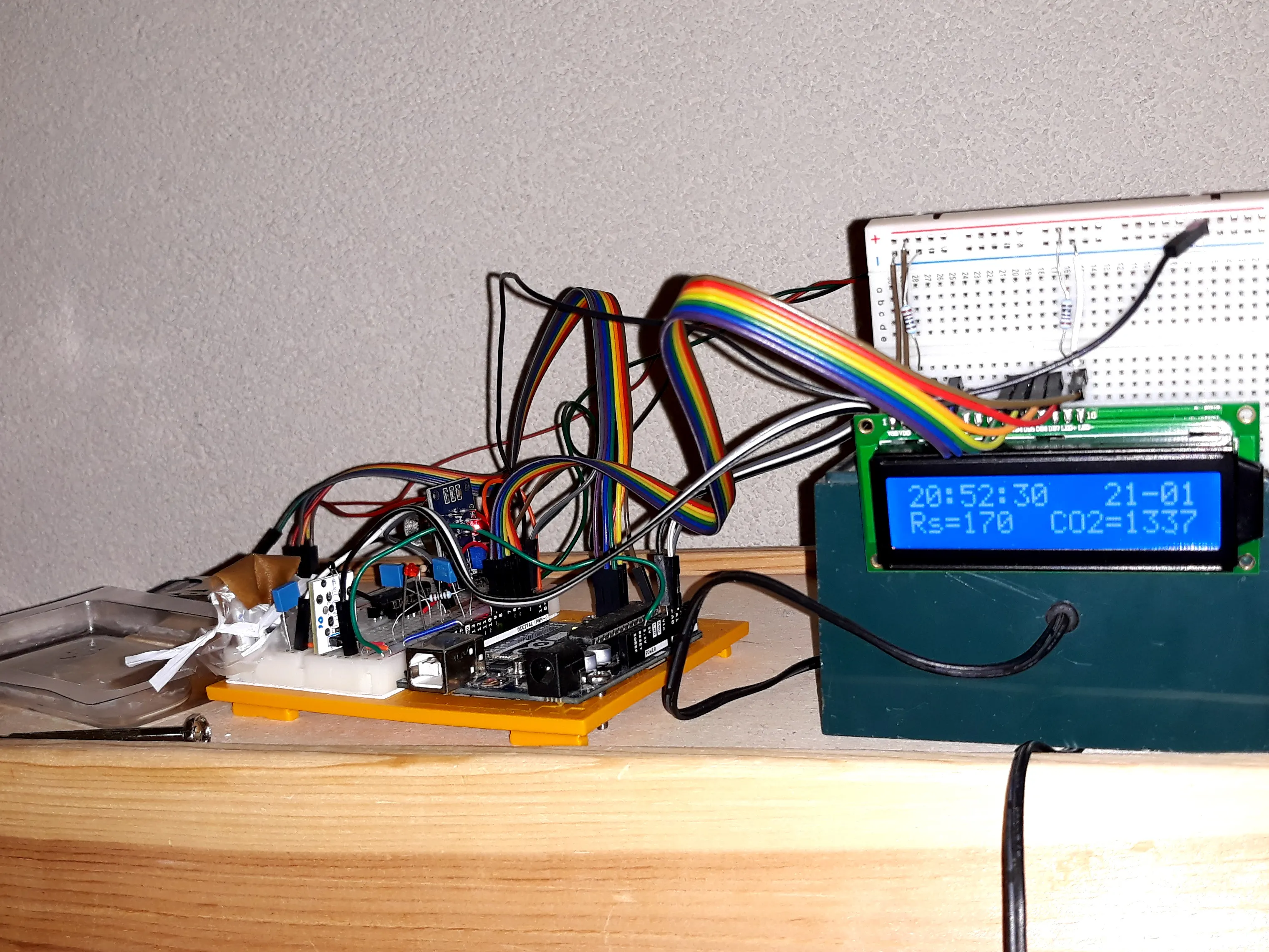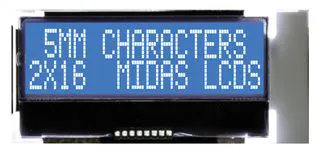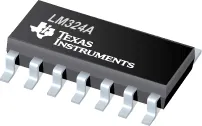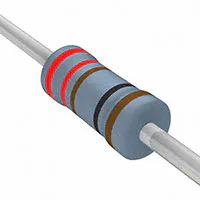# CO2 Monitor

CO2 monitor and log based on MQ-135 with temperature and relative humidity correction.

IntermediateShowcase (no instructions)3,856## Things used in this project

### Hardware componentsArduino UNO & Genuino UNO
×1Alphanumeric LCD, 16 x 2
×1Texas Instruments General Purpose Quad Op-Amp
×1
 Temperature sensor with thermistor VMA-320
×2
 MQ-135
×1
 SD card logging shield VMA-304
×1Resistor 10k ohm
×1Resistor 1k ohm
×1Resistor 221 ohm
×1

## Schematics

### Wiring CO2 measurement and logging project

Measures CO2 concentration. Correction for temperature and relative humidity. Writes results to SD card and LCD display

## Code

### CO2v2.ino

C/C++
Main program
```#include <SPI.h>
#include <SD.h>
#include <LiquidCrystal.h>
#include "Time.h"
#include "Calculations.h"

const int rs = 8, en = 7, d4 = 6, d5 = 5, d6 = 4, d7 = 3;
LiquidCrystal lcd(rs, en, d4, d5, d6, d7);

// set up variables using the SD utility library functions:
Sd2Card card;
SdVolume volume;
SdFile root;
//pin connected to CS of SD card shield
const int chipSelect = 2;

#define _dd_   20
#define _mm_    1
#define _yy_   20

#define _hr_   17
#define _min_   54
#define _sec_   0

char fileName;

//Set start time and date
Time myTime(_hr_,_min_,_sec_,_dd_,_mm_,_yy_); //hr,min,sec,d,m,y

//used connections to Arduino
const byte Td_sensor  = A0;
const byte Tw_sensor  = A1;
const byte CO2_sensor = A2;
const byte checkLight =  9;

//Data are stored in data array (double data[]) to ease printing and writing to SD card and LCD screen. Here the positions of the data in the array are given.
const byte Td   = 0; //deg Celcius
const byte Tw   = 1;
const byte RH   = 2; //relative humidity
const byte CO2  = 3; //ppm
const byte pWd  = 4; //kPa
const byte pWw  = 5;
const byte fRHT = 6; //-
const byte Rs   = 7; //kohm
const byte numData = 8; //number of data in data []

const String degC = String ("\xC2\xB0") + String("C");
const String labels[numData] = {"Td" ,"Tw" ,"RH","CO2","pWd","pWw","fRHT","Rs"  };
const String units [numData] = {degC ,degC ,"-" ,"ppm","Pa" ,"Pa" ,"-"   ,"kohm"};

void setup() {
pinMode(Td_sensor ,INPUT);
pinMode(Tw_sensor ,INPUT);
pinMode(CO2_sensor,INPUT);
pinMode(checkLight,OUTPUT);

lcd.begin(16, 2);
lcd.print("hello, world!");

Serial.begin(9600);
String fileNameConst = String("WK") + myTime.dateToString("") + String(".txt");
strcpy(fileName,fileNameConst.c_str());

Serial.print("Initializing SD card...");

// see if the card is present and can be initialized:
if (!SD.begin(chipSelect)) {
Serial.println("Card failed, or not present");
// don't do anything more:
while (1);
}
Serial.println("card initialized.");
delay(200);

// if the file is available, write to it:
// prepare datastring with current time and datalabels as column headers
myTime.updTime();
String dataString = myTime.dateToString();
dataString+= ",";
dataString+= myTime.timeToString();
for (int i = 0; i<numData; i++) {
dataString+= ",";
dataString+= labels[i];
}

File dataFile = SD.open(fileName,FILE_WRITE);
if (dataFile) {
dataFile.println();
dataFile.println(dataString);
dataFile.close();
}
// if the file isn't open, pop up an error:
else {
Serial.println("error opening datalog.txt");
}
}

void loop() {
myTime.updTime();
digitalWrite(checkLight,LOW);   //put here to see if the system is working. now not needed anymore as LCD was installed

Serial.println(myTime.timeToString() + " " + myTime.dateToString());

//fill data array with fresh measurement results
double data [numData];
data[pWd]  = calcPw(data[Td]);                 //kPa
data[pWw]  = calcPw(data[Tw]);
data[RH]   = calcRH(data[Td],data[Tw],data[pWd],data[pWw]); //dimensionless
data[fRHT] = calcfRHT(data[RH],data[Td]);      //humidity and temperature correction factor, dimensionless
data[CO2]  = calcCO2(data[Rs],data[fRHT]);     //ppm

dataToScreen(labels,data,units);

dataToLCD(labels,data);

// make a string for assembling the data to log:
String dataString = "";

dataString += ",";
dataString += myTime.timeToString();

for (int i=0; i<numData; i++) {          //add all data to dataString
dataString += ",";
dataString += String(data[i]);
}

// open the file. note that only one file can be open at a time,
// so you have to close this one before opening another.
File dataFile = SD.open(fileName, FILE_WRITE);

// if the file is available, write to it:
if (dataFile) {
dataFile.println(dataString);
dataFile.close();
}
// if the file isn't open, pop up an error:
else {
Serial.println("error opening datalog.txt");
}

//halfway time: show time, date, Rs and CO2
lcd.clear();
lcd.print(myTime.timeToString());
lcd.print("   ");
lcd.print(myTime.dateToString());
lcd.setCursor(0,1);
lcd.print("Rs=");
lcd.print(data[Rs],0);
lcd.print("  CO2=");
lcd.print(data[CO2],0);

digitalWrite(checkLight,HIGH);
const unsigned long loopDuration = 30000;
while (millis() - myTime.lastMillis() < loopDuration) { //wait until loopDuration
;
}
}

//return sensor value
int value = 0;
byte numMeas = 32; //should not be larger than 2^6=64 (int value may roll over)
for (int i=0; i<numMeas; i++) {
delay(100);  //this makes the sketch slow (32 times 100 times 3 = 9600 millisec (almost 10 sec)
}
value /= numMeas;
return value;
}

//write data to screen
void dataToScreen(const String label[],const double data[],const String unit[]) {  //all arguments declared constant as this procedure is not supposed to change any data
for (int i=0; i<numData; i++) {
if (i==RH) { //RH is printed as % instead of fraction
Serial.print(label[i]), Serial.print(":\t"), Serial.print(data[i]*100,2),  Serial.print("\t"), Serial.println("%");
}
else {
Serial.print(label[i]), Serial.print(":\t"), Serial.print(data[i],2),      Serial.print("\t"), Serial.println(unit[i]);
}
}
Serial.println();
}

//write data to LCD
void dataToLCD(const String label[],const double data[]) {
lcd.clear();
for (int i = Td; i<=CO2; i++) {
lcd.print(label[i]);
lcd.print("=");
int decimals = 1;
if (i==CO2) decimals = 0;
if (i== RH) decimals = 2;
lcd.print(data[i],decimals);
lcd.print(" ");
if (i== Td) lcd.print(" ");
if (i== Tw) lcd.setCursor(0,1);
}
}
```

### Calculations.cpp

C/C++
Calculation of temperature, relative humidity, sensor resistance based on values read from A0, A1 and A2.
From these date it calculates water vapour pressures and sensor correction factor are derived.
Finally the CO2 concentration is measured
```#include "Arduino.h"
#include "Calculations.h"

double calcTemp(int Value) {  //https://playground.arduino.cc/ComponentLib/Thermistor2/
double Temp;
Temp = log(10000.0/(1024.0/Value-1)); // for pull-up configuration
Temp = 1 / (0.001129148 + (0.000234125 + (0.0000000876741 * Temp * Temp )) * Temp );
Temp = Temp - 273.15;                // Convert Kelvin to Celcius

return Temp;//degrees C
}

double calcPw(double Temp) {  //R.C. Rodgers and G.E. Hill, Brittish Journal of Anaesthesia (1978),50, 415
const double A = 7.16728;
const double B = 1716.984;
const double C = 232.538;
double exponent = A - B/(Temp+C);
double Pw = pow(10,exponent);

return Pw;//kPa
}

double calcRH(double Td, double Tw, double pWd, double pWw) {  //https://www.1728.org/relhum.htm
double RH;
if(Tw >= Td) { //to prevent RH>1 due to measurement errors where Tw >= Td
RH = 1.0;
}
else {
double pav = 101.55; //atmospheric pressure in kPa http://www.klimaatatlas.nl/klimaatatlas.php
double N = 0.0006687451584; //kPa/K
RH  = (pWw - N*pav*(1+0.00115*Tw)*(Td-Tw))/pWd;
}

return RH; //dimensionless
}

double calcfRHT (double RH, double T) {   //Own fit from MQ-135 datasheet. It is presumed that CO2 readings are equally influenced by temperature and RH as ammonia readings in given graphs
const double Intercept = 1.782663144;
const double RC_RH     = -0.748177946;
const double RC_T      = -0.015924756;
const double RC_RHT    =  0.006677957;
double calcfRHT = Intercept + RC_RH*RH + RC_T*T + RC_RHT*RH*T;

return calcfRHT; //Rs/R0 dimensionless
}

double calcRs(int sensorReading) {   //Calculates Rs from amplified analog signal
const double gain = 1.0 + 10000.0/1000.0; //amplification of opamp as function of resistances in opamp circuit
const double Rref = 10.0; //Reference resistance (in kOhm) built in MQ-135 board
/*formula derivation:
*/

return Rsensor; //kOhm
}

double calcCO2(double Rs, double fRHT) {
const double R410    = 270.0; //Measured resistance (in kOhm) after one night with open window
const double fRHT410 = 1.08;  //calcutated correction factor after one night with open window
const double a  =  410.0;
const double b  = -2.769034857; //slope from Mad Frog

double CO2 = a*pow((Rs/fRHT)/(R410/fRHT410),b); //CO2atm = 410 ppm

return CO2;//ppm
}
```

### Calculations.h

C/C++
```#include "Arduino.h"

//this library holds all physiscs calculations

double calcTemp(int Value);

//Calculate vapour pressure from temperature
double calcPw(double Temp);

//Calculate relative humidity from wet and dry bulb temperature and vapour pressures at respective temperatures
double calcRH(double Td, double Tw, double Pwd, double Pww);

//Calculate sensitivity factor (Rs/R0) as function of relative humidity and temperature
double calcfRHT (double RH, double T);

//Calculate sensor resistance (Rs) from analog input, after signal amplification with OpAmp

//Calculate CO2 concentration from measured sensor resistance (Rs) and relative humidity and temperature correction factor
double calcCO2(double Rs,double fRHT);
```

### Time.cpp

C/C++
Time and date utility
```#include "Arduino.h"
#include "Time.h"

Time::Time (byte hr, byte mnt, byte sec, byte d, byte m, byte y) {
_timeStart = (hr*60UL+mnt)*60UL+ sec; //initialize _timeStart
_lastMillis = millis();               //last time millis() was called
_curTime = _lastMillis/1000UL;        //time since start of program in seconds (rollover after 49000 days, rollover is prevented by updDate() after 1 day)

//initialize _Time[]
_Time      = hr;
_Time      = mnt;
_Time      = sec;

//initialize _Date[]
_Date      = d;
_Date      = m;
_Date      = y;

_dayRollover  = false;
}

void Time::updTime() {
unsigned long curMillis = millis();
_curTime   += curMillis/1000UL - _lastMillis/1000UL;  //update _curTime
_lastMillis = curMillis;                              //record _lastMilles for use during next call of updTime()

unsigned long curTime = _curTime + _timeStart;
if               (curTime/(24UL*60UL*60UL) > 0) _dayRollover = true;  //if time goes beyond 23:23:59 dayrollover occurs and time returs toe
//update _Time[]
curTime      =    curTime%(24UL*60UL*60UL);
_Time     =    curTime/(60UL*60UL);
curTime      =    curTime%(60UL*60UL);
_Time     =    curTime/60UL;
curTime      =    curTime%60UL;
_Time     =    curTime;

//if needed, update _Date
if (_dayRollover) updDate(); //if dayrollover, then adjust date
}

void Time::updDate() {
const byte maxDays = {0,31,29,31,30,31,30,31,31,30,31,30,31}; //2020 is leap year, next year change num days of February
const byte day   = 0; //makes code more easy to read
const byte month = 1;
const byte year  = 2;

_Date[day]++;
if (_Date[day] > maxDays[_Date[month]]) {  //if monthrollover then adjust month
_Date[day]     = 1;
_Date[month]++;
if (_Date[month] > 12) {                 //if yearrollover then adjust year
_Date[month] = 1;
_Date[year]++;
}
}
_curTime = _curTime - (24UL*60UL*60UL);    //update _curTime (subtract one day)
_dayRollover = false;
}

unsigned long Time::lastMillis() {
return _lastMillis;
}

String Time::timeToString(String sign) {
}

String Time::dateToString(String sign) {
}

String Time::toString(byte* tostring, String sign) {
String string = "";
for (byte i=1; i<3; i++) {
string+= sign;
}
return string;
}

String string = "";
if (value < 10) {
string += "0";
}
string += String(value);
return string;
}
```

### Time.h

C/C++
```/*
*Time.h - Time library
*Records starting time and date of project (as given in source code by programmer)
*Can return String with current Time (hh:mm:ss)
*Can return String with current date (dd-mm-yy)
*Can return millis since start Time
*Created by Koen Meesters, december 2019
*Last edited Februari 2020
*/
#ifndef Time_h
#define Time_h

#include "Arduino.h"

class Time {
public:
Time(byte hr = 0, byte mnt = 0, byte sec = 0, byte d = 1, byte m = 1, byte y = 20); // date by default initialized to 1-1-2020

String timeToString(String sign = ":"); //returns time in a String, default sign for time is :
String dateToString(String sign = "-"); //returns date in a String, default sign for date is -

unsigned long lastMillis();
void updTime();                         //update the time in _Time

private:
byte _Time;                          //stores time in 3 bytes hr min sec
byte _Date;                          //stores date in 3 bytes day month year
bool _dayRollover;                      //flag set if time goes beyond 23:59:59

unsigned long _timeStart;               //start time in seconds
unsigned long _curTime;                 //current time in seconds
unsigned long _lastMillis;              //millis() last time updTime() was run

void updDate();                         //update the date in _Date (should be run at least every day, otherwise it misses 1 dayrollover)

String toString(byte*, String sign);    //makes a string from _Time or _Date